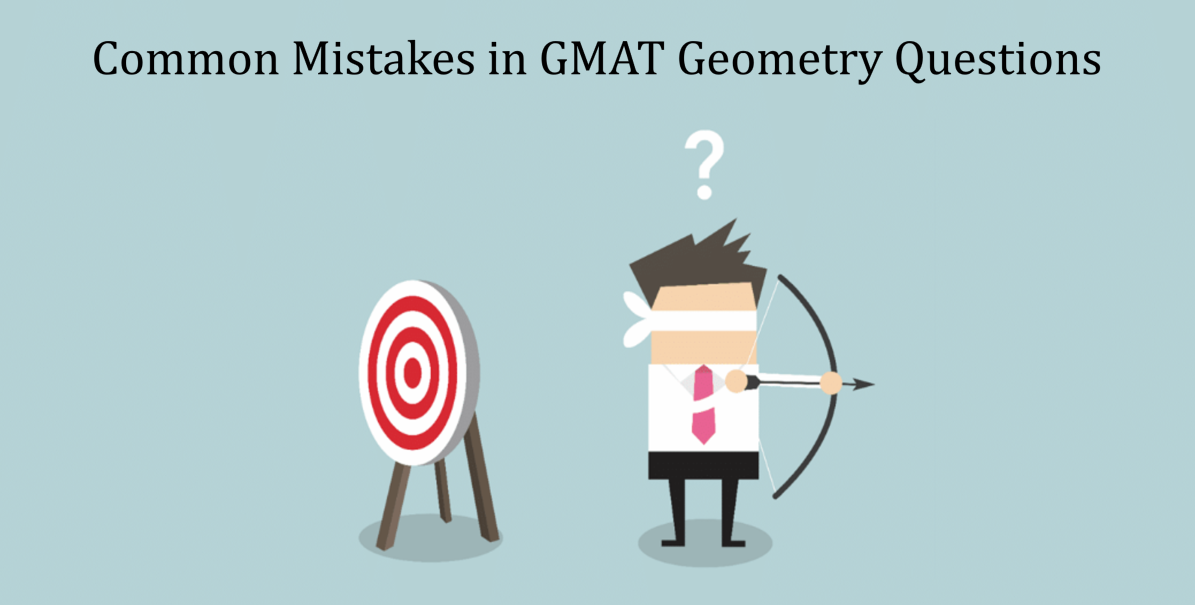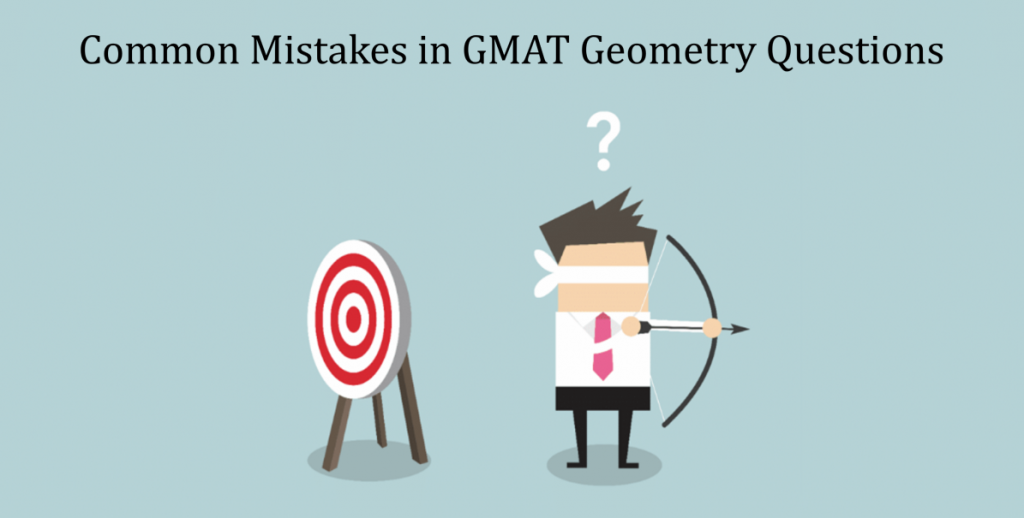Payal Tandon
Co-founder, e-GMAT
Welcome to e-GMAT Support!
I am Payal, Co-Founder of e-GMAT.
Feel free to ask any Query.We will be contacting you soon onWho wouldn’t want a Q51, V45 in GMAT? For any GMAT aspirant targeting a Q51, it is essential to avoid the Quant pitfalls. Why do I use the word ‘Pitfall’? According to the Oxford dictionary, Pitfall is a hidden or unsuspected danger or difficulty. Most students, during their GMAT Quant preparation, would make mistakes without even recognizing them. You can correct those mistakes only if you are aware of them. This article, first in the series, covers the common mistakes that students make in GMAT Geometry Questions. We will also explain

• Why students make these mistakes in GMAT Geometry Questions
• How to avoid these mistakesAnyone who is preparing for GMAT and wants to avoid common mistakes in GMAT Quant Questions should go through this series’ articles. In this series, we will focus on individual topics and sub-topics of Quant section of GMAT. We will use illustrative examples to make you aware of these pitfalls. We will also explain, how to avoid these pitfalls by refining your application of concepts. The other articles in this  series are:

## Most Common Mistakes in GMAT Geometry Questions

This is the first article of the series ‘GMAT Quant Pitfalls’ and we will focus on ‘common mistakes in GMAT Geometry Questions’. In this article, you will read about-

## Common Pitfalls – GMAT Geometry Questions

While solving Geometry questions, students tend to

1. Assume information that is not explicitly mentioned in the question.
2. Infer certain things on the basis of how the diagram “looks” – without applying basic concepts

We will use 3 illustrative examples to help you understand the above-mentioned pitfalls. For better understanding, we will recommend you to solve the questions before viewing the provided explanation.

## Illustrative Example 1

ABC is a triangle, where the length of AB = 8 cm and length of BC = 6 cm. Find the value of AC =?

1. 8 cm
2. 10 cm
3. 12 cm
4. 14 cm
5. Cannot be determined
##### Explanation

It is highly likely that some of you might have solved the question as shown below:

Since the triangle ABC is a right-angled triangle, we can find the value of AC using Pythagoras Theorem. Let’s assume the length of side AC as x.

Therefore,

x2=62+82
x2=100
(x−10)(x+10)=0

Hence the value of AC is 10. Therefore, option B seems to the correct answer.

### Pitfall Identified – Common mistakes in GMAT Geometry Questions

Hold on a moment! Before you jump to this conclusion, take a step back and think about the following questions:

1. Why did you apply Pythagoras Theorem to solve this question?
2. Is angle B really 90º?
3. Does the figure simply “look” like a right-angled triangle or is any information given about the angles of A, B, and C in the question statement?

Clearly, the question doesn’t tell us the value(s) of any of the angles A, B or C. Therefore, we cannot comment anything about their values just by “looking” at the diagram!

Hence, in this case, we cannot find the value of AC, unless we have more information about the triangle ABC.

So the correct answer to this question is Option E

Now let us see how the GMAT tests the above concept by attempting the following GMAT-like question

### Official GMAT Question – Similar to OG QR2 – 72

In the given figure, what is the value of angle ABE?

1. BD and BC are the angle bisectors of angle ABC and DBE
2. angle CBE = 45º
##### Explanation

Can you tell by just looking at the diagram, which angles are 90º?

If you are thinking that we cannot infer anything from the diagram, that’s good! You are thinking in the right direction!

### Pitfall Identified – Common mistakes in GMAT Geometry Questions

Just from the diagram, some of you might infer that angle ABC is 90º. Let us see how this affects our answer?

From statement 1 we can infer:

If we “assume” that angle ABC = 90º, and approach the problem.

Since BD is the angle bisector of ABC, angle ABD = angle DBC = 45º(since we “assumed” ABC = 90º)

Also, since BC is the angle bisector of DBE, then angle DBC = angle CBE = 45º

Hence we can get the answer from statement-I.

From the 2nd Statement we can infer:

Since CBE = 45º and we have assumed ABC = 90ºWe can write angle ABE = ABC + CBE = 135º

Hence, we can get the answer from statement-II alone as well and thus our answer would be Option D.

Is this approach correct?

No!

Correct way to solve:

Since the diagram does not have any information we will NOT assume anything.

From statement 1 :

All we can infer from the first statement is that – Angle ABD = angle DBC = angle CBE

Since we do not have the actual values, statement one is insufficient to get our answer.

From statement 2 :

Angle CBE = 45º is given. But that cannot help in finding angle ABE.

Hence statement 2 is also insufficient to get our answer.

Combining both the statements

Since, Angle ABD = angle DBC = angle CBE and CBE = 45º, we can infer that Angle ABD = angle DBC = angle CBE = 45º

Angle ABE = angle (ABD + DBC + CBE) = 135º

Notice that the incorrect assumption leads you to the same value for the angle as the official solution does (i.e. angle ABE = 135º).

However, when that information is assumed beforehand, we arrive at the incorrect conclusion that we can get the answer from either of the statements. This is incorrect since to arrive at the answer, both statements together need to be taken into account.

The devil is in the details! Planning out the finer details of your study schedule takes you one step closer to your target GMAT score. Learn the other benefits of a well defined study plan in this article.

Save 60+ hours of GMAT preparation by crafting a well-defined study plan in just 3 steps:

## Illustrative Example 2 – Geometry Question

ABCD is a quadrilateral as shown in the figure given below, Find the area of the quadrilateral ABCD.

1. The diagonals bisect each other at 900
2. The length of one of the sides is 10 cm.

### Explanation:

Many of you would think that both the statements together are sufficient to answer this Data Sufficiency question.

First, you’d infer that since the diagonals are perpendicular and bisect each other, the quadrilateral is either a square or a rhombus. (if you could infer this, then good job! You have started off on the right note.)

### Pitfall Identified – Common mistakes in GMAT Geometry Questions

After this inference, many test takers usually end up making the following assumption:

• The angles A, B, C and D are 90º (based on the way the diagram “looks”)

We can sum up the subsequent analysis as:

Since all angles are 90º, the figure cannot be a rhombus. Therefore, it is a square and the area is Side2=102=100

These mistakes are very common among students, especially when they don’t remember the basic properties of a triangle or a quadrilateral. Hence, there is a natural tendency of assuming information that is not present in the question by just looking at the diagram.

This approach is INCORRECT and costs a lot in the examination.

In this question, we cannot find the answer and therefore, the correct option is actually option E.

Let’s see why:

1. We can only infer that the quadrilateral could be a square or a rhombus
2. If we know that diagonals bisect each other at right angles and they are equal in length, then we could have concluded that the quadrilateral is a square!

• In case of a rhombus, the diagonals bisect each other at right angles, but they are not equal
• Since we cannot say for sure whether it is square or a rhombus, therefore, we cannot find out exactly, what is the area of this quadrilateral

GMAT might tweak the question and present the data in the following way also to test us on the above concept –

Consider the above figure with given data – ABCD is a quadrilateral. Find the area of the quadrilateral –

1. The diagonals bisect each other at 90º and they are equal.
2. The length of each diagonal is 10 cm.

Can you tell me what will be the answer in this case?

## Illustrative Example 3 – Geometry Question

A circle circumscribes the triangle ABC and length of the three sides of the triangle are 8 cm, 15 cm and 17 cm respectively.

Find out the radius of the circle.

1. 4.5
2. 7.5
3. 8.5
4. 10.5
5. Cannot be determined
##### Approach:

Observe the lengths of the triangle ABC carefully. Can we infer that ABC is a right angle triangle? Yes, we can!

Because it satisfies the Pythagoras theorem.

Hypotenuse2=Perpendicular2 + Base2

172 = 152 + 82

Note that the above inference cannot be arrived at just by looking at the diagram

From our analysis, we can infer that ABC is a right angled triangle. Let us redraw the diagram using the above inference. Notice that in the diagram I did not mark the points A, B and C, as I do not know which lengths are AB, BC or CA

But is it important for us to know that? No, it’s not.

If you know the following property of a circle –

“The diameter of a circle subtends a right angle to any point on the circle.”

You can easily conclude that 17 is the diameter of the circle. And since Radius = Diameter/2

The radius of the circle = 17/2 = 8.5

## Takeaways

Hence you can see from the above examples, that it is not a good practice to draw inferences by just looking at the diagram. Never assume anything from the figure, unless

1. It is explicitly stated in the question statement or figure OR
2. It can be deduced from the given information in the question or figure

The proper approach to avoid Common Mistakes in GMAT Geometry Questions should be :

1. Observe the given data carefully
2. Derive inferences from the given data
3. Then use those inferences along with the given diagram to get to the final solution.

Keeping these takeaways in mind, can you solve the next 4 Geometry questions provided below?

Make sure to present your analysis for these questions. Happy Learning!

If you are planning to take the GMAT, we can help you with a personalized study plan and give you access to quality online content to prepare. Write to us at acethegmat@e-gmat.com. We are the most reviewed GMAT prep company on gmatclub with more than 2400 reviews and are the only prep company that has delivered more than 700+ scores than any other GMAT club partner. Why don’t you take a free trial and judge for yourself?

GMAT Geometry – Practice Questions:#### Suheb Hussain

###### Browse Related Topics# Achieve 740+on the GMAT in 30 days!